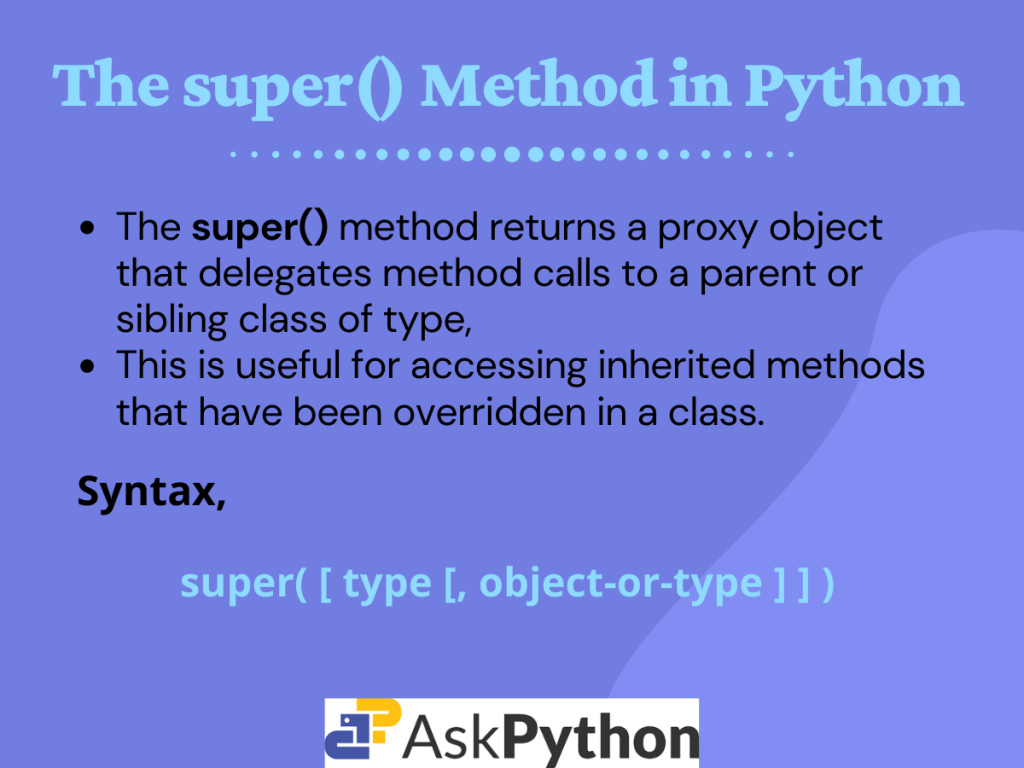# Understanding the super() Method in Python## Introduction

Today in this tutorial, we are going to discuss the super() method in Python.

Before diving right into the topic, we highly recommend going through the tutorial on Python Inheritance.

Once you know about inheritance in Python, we can get started.

## The super() Method in Python

The `super` method returns a proxy object that delegates method calls to a parent or sibling class of type. This is useful for accessing inherited methods that have been overridden in a class.

Or simply, it is used to call the constructor, i.e. the` __init__()` method of the superclass.

Syntax for using super in Python is given below.

```super([type[, object-or-type]])
```

In Python 3.x versions, we can use super without passing the above two parameters. Look at the code snippet below.

```class C(B):
def method(self, arg):
super().method(arg)
```

Here, `C` is the derived class, `B` is the base class, method is a user defined function with argument `arg`.

As you can see, the line `super().method(arg)` is actually equivalent to `super( C, self).method(arg)` in Python 3.x. This is not allowed in Python 2.x. Hence, using super there is a bit tricky.

## Using super()

Consider the below given example.

```class Demo:
a = 0
b = 0
c = 0
def __init__(self,A,B,C):
self.a = A
self.b = B
self.c = C
def display(self):
print(self.a, self.b, self.c)

class Newdemo(Demo):
d = 0
def __init__(self,A,B,C,D):
self.a = A
self.b = B
self.c = C
self.d = D

def display(self):
print(self.a, self.b, self.c,self.d)

B1 = Demo(100,200,300)
print("Contents of Base Class:")
B1.display()
D1 = Newdemo(10,20,30,40)
print("Contents of Derived Class:")
D1.display()
```

Output:

In the above example, the classes derived from the base class `Demo` were not implemented efficiently or robustly.

The derived class `Newdemo` explicitly initializes the value of A, B, and C fields of the base class. The same duplication of code is found while initialization of the same fields in the base class, `Demo` too.

This process is inefficient. This implies that a subclass must be granted access to the members of a superclass.

Therefore, whenever a subclass needs to refer to its immediate super class, `super` comes into the picture.

### Super() to Call Super Class Constructor

Now let us apply the `super()` method for the above example.

```class Demo:
a = 0
b = 0
c = 0
def __init__(self,A,B,C):
self.a = A
self.b = B
self.c = C
def display(self):
print(self.a, self.b, self.c)

class Newdemo(Demo):
d = 0
def __init__(self,A,B,C,D):
self.d = D
super().__init__(A,B,C) #super to call super Class
#The __init__() Method

def display(self):
print(self.a, self.b, self.c,self.d)

B1 = Demo(12,34,56)
print("Contents of Base Class:")
B1.display()
D1 = Newdemo(11,22,33,44)
print("Contents of Derived Class:")
D1.display()
```

Output:

Here, the derived class `Newdemo` calls the `super()` with arguments a, b, and c. This causes the constructor `__init__` of the base class, i.e. `Demo` to be called. This initialises the values of a, b, and c. Hence, the `Newdemo` class no longer initialises the values itself.

## Using super in Python 2.x

The syntax to call a `super` class constructor in Python 2.x is given below,

```super(Derived_Class_Name, self).__init__(Parameters_of_Super_Class_Constructor)
```

Hence we need to make some minor changes to the above example if we want to use it in Python 2.

Firstly, we need to put `object` in the base class as shown below.

```class Demo(object):
...#other statements
```

And secondly, pass `Newdemo` and `self` at the site of super class call. Like this.

```super(Newdemo,self).__init__(A,B,C) #super to call super Class
...        #The __init__() Method
```

## Why we need super()

In the case of single inheritance with a parent and offspring class, the `super` function is used to implicitly refer to the parent class without naming it explicitly. This makes the code more efficient, maintainable and robust in nature.

Nextly, for a multi-level inheritance, the `super` method can be used to refer to the immediate superclass implicitly. This again makes the code easier to understand and highly maintainable.

## Conclusion

So in this tutorial we understood the concept of super() method in Python, its use and need.

For any further questions, feel free to comment below.